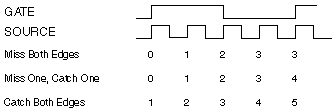# Quantization Error

Quantization error is the inherent uncertainty in digitizing an analog value as a result of the finite resolution of the conversion process. Quantization error depends on the number of bits in the converter, along with its errors, noise, and nonlinearities. Quantization error occurs due to phase differences between the input signal and the counter timebase. Depending on how the phase of the input signal and counter timebase align, the count measured has three possibilities:

• Miss Both Edges—The counter recognizes neither the first rising edge nor the last rising edge of the counter timebase, giving a count of one less than the expected value.
• Miss One, Catch One—The counter only recognizes the first rising edge or the last rising edge of the counter timebase, giving the expected value.
• Catch Both Edges—The counter recognizes both the first rising edge and the last rising edge of the counter timebase, giving a count of one more than the expected value.For example, if the counter timebase rate is 20 MHz, and the frequency of the input signal is 5 MHz, the measured value can be 3, 4, or 5 due to quantization error. This corresponds to a measured frequency of 6.67 MHz, 5 MHz, or 4 MHz, resulting in a quantization error of as much as 33%.

### Quantization Error with One Counter Time Measurements

For one counter time measurements, the following equation gives the quantization error.

ErrQuantization = Actual Frequency / (Counter Timebase Rate - Actual Frequency)

You can reduce the quantization error for single counter time measurements by increasing the counter timebase rate. The following table shows the quantization error for various timebase rates with given input signal frequencies:

Actual Frequency of Input Signal Counter Timebase Rate Quantization Error
10 Hz 100 kHz 0.01%
100 Hz 100 kHz 0.10%
1 kHz 100 kHz 1.01%
10 kHz 100 kHz 11.11%
10 kHz 20 MHz 0.05%
100 kHz 20 MHz 0.50%
1 MHz 20 MHz 5.26%
2 MHz 20 MHz 11.11%
5 MHz 20 MHz 33.33%

For period and frequency measurements, if the quantization error is too large for your input signal, you might consider using one of the two counter period and frequency measurements.

### Quantization Error with High Frequency Two Counter Method

For two counter high-frequency measurements, the following equations give the quantization error.

ErrQuantization = Actual Period / Measurement Time

ErrQuantization = 1 / (Measurement Time × Actual Frequency)

Increasing the measurement time reduces the quantization error. The quantization error also decreases with higher frequency input signals. The following table shows the quantization error for various measurement times and input signal frequencies:

Actual Frequency of Input Signal Measurement Time Quantization Error
10 kHz 1 ms 10.00%
100 kHz 1 ms 1.00%
1 MHz 1 ms 0.10%
5 MHz 1 ms 0.02%
10 MHz 1 ms 0.01%
10 kHz 10 ms 1.00%
100 kHz 10 ms 0.10%
1 MHz 10 ms 0.01%
5 MHz 10 ms 0.002%
10 MHz 10 ms 0.001%
10 kHz 100 ms 0.10%
100 kHz 100 ms 0.010%
1 MHz 100 ms 0.001%
5 MHz 100 ms 0.0002%
10 MHz 100 ms 0.0001%
10 kHz 1 s 0.010%
100 kHz 1 s 0.0010%
1 MHz 1 s 0.0001%
5 MHz 1 s 0.00002%
10 MHz 1 s 0.00001%

As the table shows, quantization error is reduced at higher frequencies of the input signal. However, the advantage of this measurement method disappears at lower frequency input signals because you need to measure longer to gain accuracy, and you use up more resources.

### Quantization Error with Large Range Two-Counter Measurement Method

For two counter large-range measurements, the following equations give the quantization error.

ErrQuantization = 1 / (Divisor × Counter Timebase Rate × Actual Period – 1)

ErrQuantization = Actual Frequency / (Divisor × Counter Timebase Rate – Actual Frequency)

Increasing the divisor, increasing the counter timebase rate, or lowering the input signal frequency reduces the quantization error. The table lists the quantization error for various divisors and input signal frequencies assuming a counter timebase rate of 20 MHz.

Actual Frequency of Input Signal Divisor Quantization Error
1 kHz 4 0.00125%
100 kHz 4 0.125%
1 MHz 4 1.266%
1 kHz 10 0.0005%
100 kHz 10 0.05%
1 MHz 10 0.5%
1 kHz 100 0.00005%
100 kHz 100 0.005%
1 MHz 100 0.05%

Notice that the use of a divisor reduces the quantization error. Although the high frequency two-counter measurement method is more accurate at higher frequencies, the large range two-counter measurement method is more accurate throughout the range in a shorter amount of time. For example, if the input signal varies between 1 kHz and 1 MHz and you require a maximum quantization error of 2.0% at any signal range, you need a minimum measurement time of 50 ms using the high frequency two-counter measurement method. To gain the same accuracy using the large range two-counter method requires a maximum measurement time of 4 ms for any one measurement.